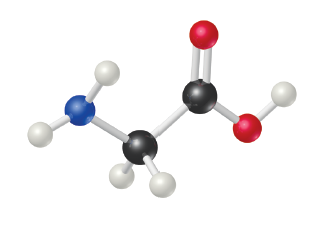# Problem: Glycine, an amino acid used by organisms to make proteins, is represented by the molecular model below.Calculate the percent nitrogen by mass in glycine.

🤓 Based on our data, we think this question is relevant for Professor Czader's class at UH.

###### FREE Expert Solution

We’re asked to calculate the percent nitrogen by mass in glycine based on its molecular model.

Recall the mass percent formula:

The  %N by mass in glycine can be calculated using the equation:

To do so, we need to determine the molecular formula of glycine and its molar mass.

First, we need to identify the element represented by each sphere based on its color.

Recall the color schemes used for a molecular model:

• white = Hydrogen, H
• red = Oxygen, O
• blue = Nitrogen, N
• black = Carbon, C###### Problem Details

Glycine, an amino acid used by organisms to make proteins, is represented by the molecular model below.Calculate the percent nitrogen by mass in glycine.